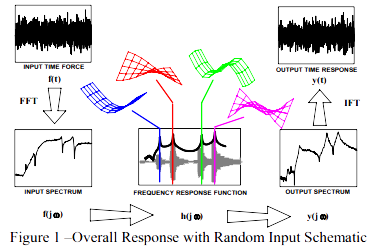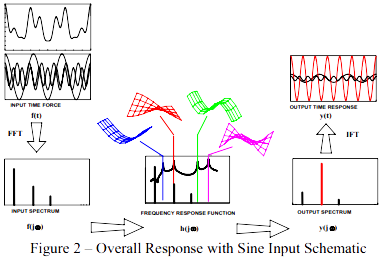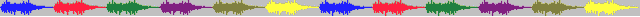# 52.模态空间06.08-什么是好的MAC值，这样我就知道模型正确与否了？

### 模态空间 – 在我们自己的小世界中     Pete Avitabile(著) KSI科尚仪器 董书伟（译）#### 我们讨论一下这个问题。

2014年05月10日 发布 ver1.0FRF和脉冲响应不过是加到输入激励上的一种滤波器。显示了FRF，同时显示了各个固有频率上的相应模态振型。所以我们看得出，频率和模态振型对确定系统的响应是非常重要的。尽管振型正确，但频率误差也很重要。

$\left [ M \right ]\left \{ \ddot{x} \right \}+\left [ C \right ]\left \{ \dot{x} \right \}+\left [ K \right ]\left \{ x \right \}=\left \{ F\left ( t \right ) \right \}$

$\begin{bmatrix} \setminus & & \\ &\bar{M} & \\ & &\setminus \end{bmatrix}\left \{ \ddot{p} \right \}+\begin{bmatrix} \setminus & & \\ &\bar{C} & \\ & &\setminus \end{bmatrix}\left \{ \dot{p} \right \}+\begin{bmatrix} \setminus & & \\ &\bar{K} & \\ & &\setminus \end{bmatrix}\left \{ p \right \}=\left [ U \right ]^{T}\left \{ F \right \}$《振动：解析与试验模态分析》辛辛那提大学教授Randall J. Allemang博士 编著 KSI科尚仪器 董书伟 翻译…